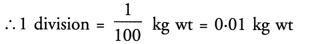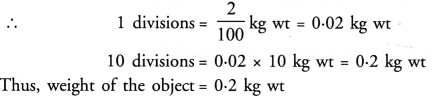## Value Based Questions in Science for Class 9 Chapter 10 Gravitation

These Solutions are part of Value Based Questions in Science for Class 9. Here we have given Value Based Questions in Science for Class 9 Chapter 10 Gravitation

Question 1.
A spring balance for measuring weight of the range 0-0.5 kg wt has total 10 divisions on its scale. What is the least count of the spring balance ?
Least count of the spring balance = Value of 1 division on its scale.
Here, 10 divisions = 0.5 kg wtTherefore, least count of the spring balance = 0.05 kg wt.

More Resources

Question 2.
A spring balance of the range 0-1 kg wt has total 100 divisions on its scale. What is the least count of the spring balance ?